# Buổi 2 - Quantitative - Reading 8: Probability Concept & Reading 9: Common probability distributions

READING 8: PROBABILITY CONCEPT

Question 8.1: Which of the following is an a priori probability ?
A) For a stock, based on prior patterns of up and down days, the probability of the stock having a down day tomorrow.
B) On a random draw, the probability of choosing a stock of a particular industry from the S&P 500.
C) The probability the Fed will lower interest rates prior to the end of the year.
Explanation
B is correct. A priori probability is based on formal reasoning and inspection. Given the number of stocks in the airline industry in the S&P500 for example, the a priori probability of selecting an airline stock would be that number divided by 500

Question 8.2:
If the odds against an event occurring are twelve to one, what is the probability that it will occur?
A) 0.0833.
B) 0.9231.
C) 0.0769.
Explanation
The way odds are stated: odds against = 1 - P(E)/P(E)
Odds against = 12 => P (E) = 1/13 = 0.0769 => C is correct.

Question 8.3: Helen Pedersen has all her money invested in either of two mutual funds (A and B). She knows that there is a 40% probability that fund A will rise in price and a 60% chance that fund B will rise in price if fund A rises in price. What is the probability that both fund A and fund B will rise in price?
A) 1.00.
B) 0.24.
C) 0.40
Explanation
Multiplication rule of possibility: P (AB) = P (BA) = P (B|A) x P (A) = P (A|B) x P (B)
P (A) = 0.40, P (B|A) = 0.60. Therefore, P (AB) = P (A) x P (B|A) = 0.40(0.60) = 0.24.

Question 8.4: If the probability of event J multiplied by the probability of event K is not equal to the joint probability of events J and K, then events J and K are most likely:

A) dependent events.
B) independent events.
C) mutually exclusive events.

Explanation
A is correct.

• Events J and K are dependent. By the multiplication rule, joint probability P(JK) = P(J|K) × P(K), or P(JK) = P(K|J) × P(J).
• Events are independent if P(J|K) = P(J) and P(K|J) = P(K). This implies that for independent events, P(JK) = P(J) × P(K). If this condition is not met, events J and K are dependent. If events J and K are mutually exclusive, their joint probability is zero.
• The information given is consistent with this but not sufficient to conclude that this is the case.

Question 8.5: Assume two stocks are perfectly negatively correlated. Stock A has a standard deviation of 10.2% and stock B has a standard deviation of 13.9%. What is the standard deviation of the portfolio if 75% is invested in A and 25% in B?
A) 0.00%.
B) 4.18%.
C) 0.17%

Explanation
B is correct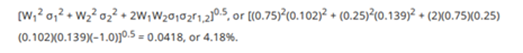Question 8.6: A parking lot has 100 red and blue cars in it.

40% of the cars are red.

70% of the red cars have radios.

80% of the blue cars have radios.

What is the probability that the car is red given that it has a radio?
A) 28%.
B) 47%.
C) 37%

Explanation
Suppose:

P (A): probability that a car has a radio
P (B): probability that a car is red
=> the probability that the car is red given that it has a radio: P (B|A)
P (B|A) = P (A|B) x P(B) : P(A)
Step 1: Calculated P(A)
Using total probability rule, P(A) = 40% x 70% + 60% x 80% = 76%
Step 2: Calculated P(B|A)
P(B|A) = P (A|B) x P(B) : P(A) = 40% x 70% : 76% = 36.84% => C is correct

Question 8.7: The covariance of the returns on investments X and Y is 18.17. The standard deviation of returns on X is 7%, and the standard deviation of returns on Y is 4%. What is the value of the correlation coefficient for returns on investments X and Y?

A) +0.65.
B) +0.32.
C) +0.85.

Explanation

The correlation coefficient = Cov (X, Y) / [(Std Dev. X) (Std. Dev. Y)] = 18.17 / 28 = 0.65 => A iss correct

Question 8.8:
The covariance of returns is positive when the returns on two assets tend to:
A) have the same expected values.
B) be above their expected value at different times.
C) be on the same side of their expected value at the same time.
Explanation
C is correct. The covariance of returns is positive when the returns on both assets tend to be on the same side (above or below) their expected values at the same time, indicating an average positive relationship between returns.

Question 8.9: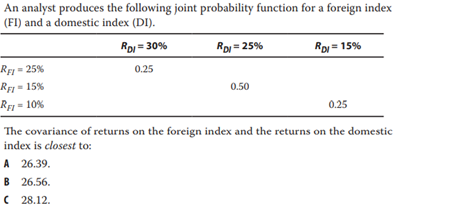Explanation
Step 1: Calculate the exxpected return off each index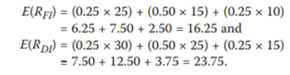Step 2: Calculated the covariance between 2 stocks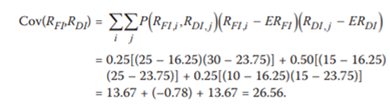Question 8.10: A Firm is going to create three teams of four from twelve employees. How many ways can the twelve employees be selected for the three teams?
A) 495.
B) 34,650.
C) 1,320.
Explanation
B is correct. This problem is a labeling problem where the 12 employees will be assigned one of three labels. It requires the labeling formula. There are [(12!) / (4! × 4! × 4!)] = 34,650 ways to group the employees.

READING 9: COMMON PROBABILITY DISTRIBUTIONS

Question 9.1: Which of the following is least likely to be an example of a discrete random variable?
A) The number of days of sunshine in the month of May 2006 in a particular city.
B) The rate of return on a real estate investment.
C) Quoted stock prices on the NASDAQ.
Explanation
B is correct.The rate of return on a real estate investment, or any other investment, is an example of a continuous random variable because the possible outcomes of rates of return are infinite (e.g., 10.0%, 10.01%, 10.001%, etc. Both of the other choices are measurable
(countable).

Question 9.2: Which of the following statements about common probability distributions is least accurate?
A) A probability distribution specifies the probabilities of the possible outcomes of a random variable.
B) In a binomial probability distribution, each observation has only two possible outcomes that are mutually exclusive.
C) normal distribution is a discrete symmetric probability distribution that is completely described by two parameters: its mean and variance.
Explanation
C is correct. A normal distribution is a continuous symmetric probability distribution.

Question 9.3: Consider a random variable X that follows a continuous uniform distribution: 7 ≤ X ≤ 20. Which of the following statements is least accurate?
A) F(12 ≤ X ≤ 16) = 0.307.
B) F(21) = 0.00.
C) F(10) = 0.23
Explanation
B is correct. F(21) = 1.00. For a cumulative distribution function, the expression F(x) refers to the probability of an outcome less than or equal to x. In this distribution all the possible outcomes are between 7 and 20. Therefore the probability of an outcome less than or equal to 21 is 100%.

Question 9.4: Which of the following is NOT an assumption of the binomial distribution?
A) Random variable X is discrete.
B) The expected value is a whole number.
C) The trials are independent.
Explanation
B is correct. The expected value is n × p. A simple example shows us that the expected value does not have to be a whole number: n = 5, p = 0.5, n × p = 2.5. The other conditions are necessary for the binomial distribution.

Question 9.5: A portfolio manager annually outperforms her benchmark 60% of the time.
Assuming independent annual trials, what is the probability that she will outperform her benchmark four or more times over the next five years?
A) 0.26
B) 0.34
C) 0.48
Explanation
=> B is correct.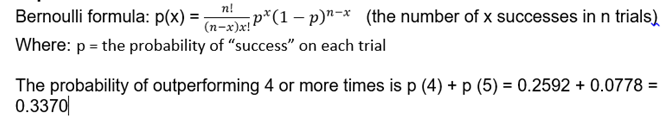Question 9.6: The farthest point on the left side of the lognormal distribution:
A) is bounded by 0.
B) can be any negative number.
C) is skewed to the left.
Explanation
A is correct.The lognormal distribution is skewed to the right with a long right hand tail and is bounded on the left hand side of the curve by zero.

Question 9.7: An analyst is creating a binomial tree for a stock price based on the following assumptions: the probability of an up move is 62%, the up factor is 1.15, and the down factor is 1/1.15. The probability that the stock price will be unchanged after two periods is closest to:
A) 24%.
B) 42%.
C) 47%.
Explanation
C is correct.The stock price is unchanged after 1 up move and 1 down move
=> The probability that the stock price will be unchanged is 2 x 62% x 38% = 47.12% (up-down, down – up)

Question 9.8: If a stock's return is normally distributed with a mean of 16% and a standard deviation of 50%, what is the probability of a negative return in a given year?
A) 0.3745.
B) 0.5000.
C) 0.0001.
Explanation
A is correct. The selected random value is standardized (its z-value is calculated) by subtracting the mean from the selected value and dividing by the standard deviation. This results in a z-value of (0 – 16) / 50 = -0.32. Changing the sign and looking up +0.32 in the z-value table yields 0.6255 as the probability that a random variable is to the right of the standardized value (i.e. more than zero). Accordingly, the probability of a random variable being to the left of the standardized value (i.e. less than zero) is 1 – 0.6255 = 0.3745.

Question 9.9: Which of the following statements about the normal distribution is least accurate?
A) The normal distribution: has a mean of zero and a standard deviation of one.
B) is completely described by its mean and standard deviation.
C) is bell-shaped, with tails extending without limit to the left and to the right.
Explanation
A is correct. The standard normal distribution has a mean of 0 and a standard deviation of 1.

Question 9.10: A stated interest rate of 9% compounded continuously results in an effective annual rate closest to:
A) 9.20%.
B) 9.42%.
C) 9.67%.
Explanation
B is correct. The effective annual rate with continuous compounding = e^Rcc – 1 = e^0.09 – 1 = 0.09417, or 9.42%

Question 9.11: A Monte Carlo simulation can be used to:
A) directly provide precise valuations of call options.
B) simulate a process from historical records of returns.
C) test the sensitivity of a model to changes in assumptions
Explanation
C is correct. A characteristic feature of Monte Carlo simulation is the generation of a large number of random samples from a specified probability distribution or distributions to represent the role of risk in the system.